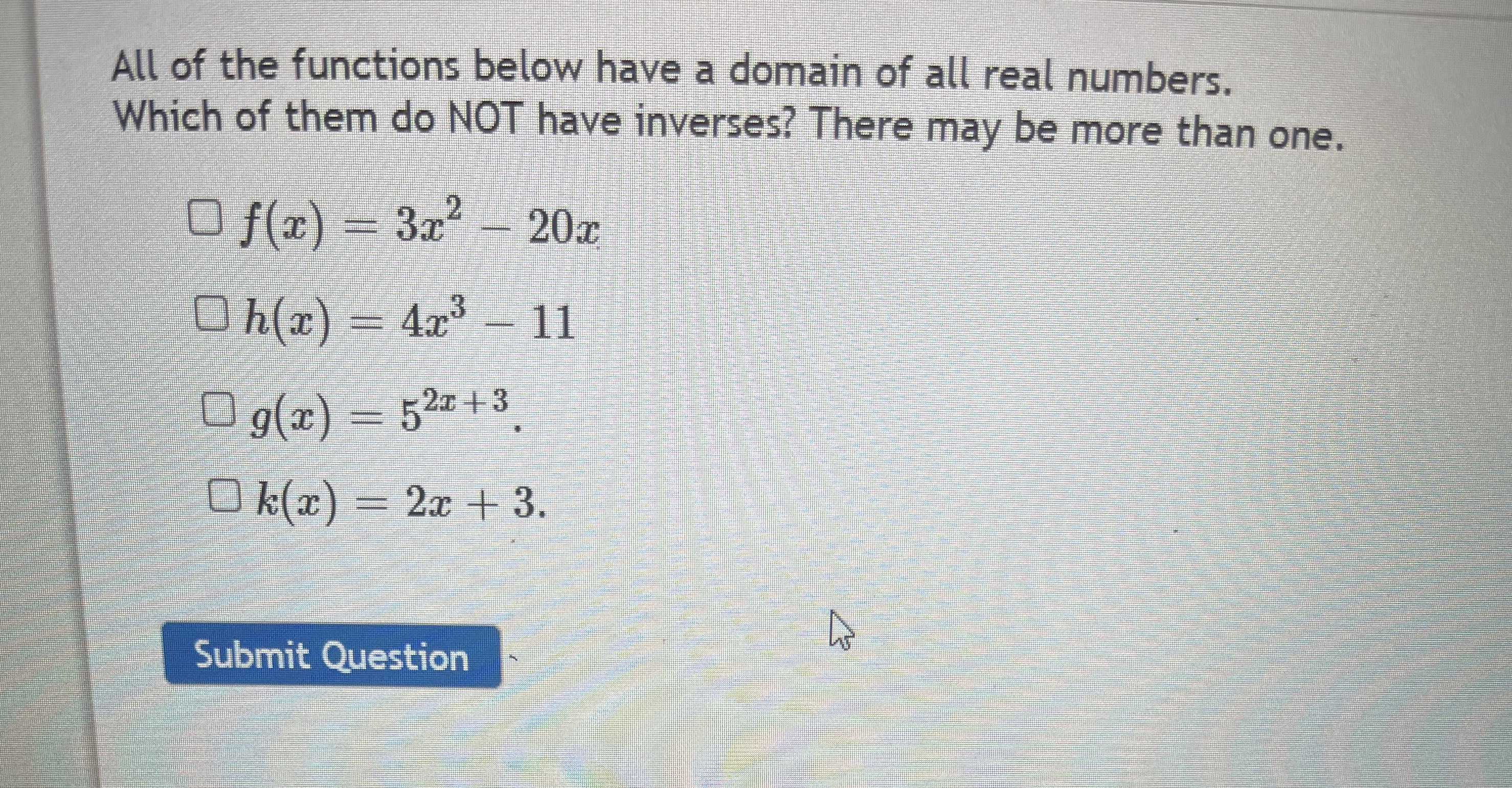### Still have math questions?

Algebra
QuestionAll of the functions below have a domain of all real numbers. Which of them do NOT have inverses? There may be more than one.

$$\square f ( x ) = 3 x ^ { 2 } - 20 x$$

$$\square h ( x ) = 4 x ^ { 3 } - 11$$

$$\square g ( x ) = 5 ^ { 2 x + 3 }$$

$$\square k ( x ) = 2 x + 3$$ . Submit Question